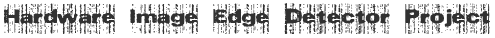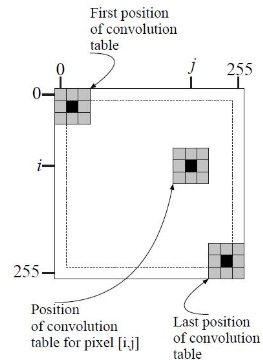Edge Detection in FPGA using Sobel Operator

### Sobel Edge Detection Operator

The Sobel Edge Detection Operator is a 3x3 Spatial mask. It is based on the differential operation [1 0 -1] and an averaging operator [1 2 1], Convolving these operators we get the 3x3 spatial mask for sobel:

```-1 0 1      Horizontal Gradient Operator
-2 0 2
-1 0 1
```
```-1 -2 -1    Vertical Gradient Operator
0  0  0
1  2  1
```The Spatial mask is convolved over the image to obtain the edge or high passed image. The image shows the convolution mask working over an image. The mask is convolved and the center element is replaced as the mask operates on the image. The borders of the images are usually blacked out, since they cannot be computed with a 3x3 mask, or partially computed.

```Gx[i, j] = Im[i+1, j-1] + 2*Im[i+1, j] + Im(i+1, j+1) �
{ Im[i-1, j-1] + 2*Im[i-1, j] + Im(i-1, j+1) }

Gy[i, j] = Im[i-1, j+1] + 2*Im[i, j+1] + Im(i+1, j+1) �
{ Im[i-1, j-1] + 2*Im[i, j-1] + Im(i+1, j-1) }
```

Tags: Edge Detection , Digital Image Processing , Sobel ,